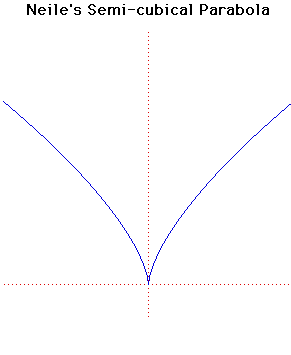# Neile's Semi-cubical ParabolaCartesian equation:
y3 = a x2

Click below to see one of the Associated curves.

Click THIS LINK to experiment interactively with this curve and its associated curves.

This curve, sometimes called the semi-cubical parabola, was discovered by William Neile in 1657. It was the first algebraic curve to have its arc length computed. Wallis published the method in 1659 giving Neile the credit. The Dutch writer Van Heuraet used the curve for a more general construction.

William Neile was born at Bishopsthrope in 1637. He was a pupil of Wallis and showed great promise. Neile's parabola was the first algebraic curve to have its arc length calculated; only the arc lengths of transcendental curves such as the cycloid and the logarithmic spiral had been calculated before this. Unfortunately Neile died at a young age in 1670 before he had achieved many further results.

In 1687 Leibniz asked for the curve along which a particle may descend under gravity so that it moves equal vertical distances in equal times. Huygens showed that the semi-cubical parabola x3 = ay2 satisfied this property. Because of this it is an isochronous curve.

The semi-cubical parabola is the evolute of a parabola.

Other Web site:

JOC/EFR/BS January 1997